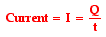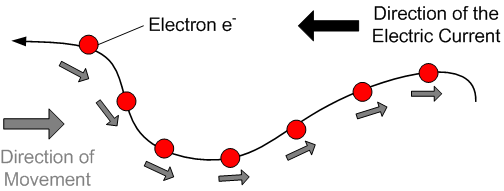Electric Current - Electrical Engineering Gate

# Electrical Engineering Gate

Online Electrical Engineering Study Site

## Friday, October 10, 2014

Electric current is the rate of charge flow past a given point in an electric circuit, measured in Coulombs/second which is named Amperes. In most DC electric circuits, it can be assumed that the resistance to current flow is a constant so that the current in the circuit is related to voltage and resistance by Ohm's law. The standard abbreviations for the units are
1 A = 1C/s

Thus, current as a rate quantity would be expressed mathematically asNote that the equation above uses the symbol I to represent the quantity current
As is the usual case, when a quantity is introduced in The Physics Classroom, the standard metric unit used to express that quantity is introduced as well. The standard metric unit for current is the ampere. Ampere is often shortened to Amp and is abbreviated by the unit symbol A. A current of 1 ampere means that there is 1 coulomb of charge passing through a cross section of a wire every 1 second
1 ampere = 1 coulomb / 1 second

The direction of the currentthe direction of the current in electric circuits is taken as the direction of positive charge flow, the direction opposite to the actual electron drift. When so defined the current is called conventional current